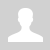Simplification logic expressions

11.3.3.1 distinguish between laws of Boolean logic

11.3.3.2 simplify logical expressions using the laws of Boolean logic

Simplification logic expressions

Logic laws

The list of rules used for the boolean expression simplifications.

Name Logic laws

The Idempotent Laws

A * A = A

A + A = A

The Identity Laws

A * 0 = 0

A * 1 = A

A + 0 = A

A + 1 = 1

The Involution Law

A = A
The Complement Laws

A * A = 0

A + A = 1

The Commutative Laws

A * B = B * A

A + B = B + A

The Associative Laws

(A * B) * C = A * (B * C)

(A + B) + C = A + (B + C)

The Distributive Laws

A * (B + C) = A * B + A * C

A + B * C = (A + B) * (A + C)

Consensus theorem

A + A * B = A + B

A + A * B = A + B

A + A * B = A + B

A + A * B = A + B

De Morgan's laws

A * B = A + B

A + B = A * B

!!! A * B + A * C = A * B + A * C + B * C

Examples of simplification:

Example 1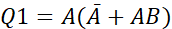- apply consensus theorem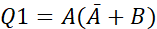- apply the distributive law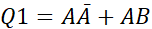- apply the complement law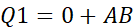- apply the identity law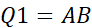Example 2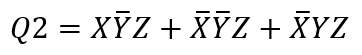- apply the distributive law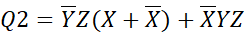-  apply the complement law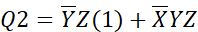- apply the identity law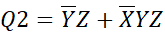- apply the distributive law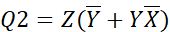- apply consensus theorem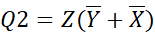- apply the distributive law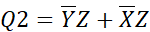Questions:

Exercises:

Ex. 1 (Author: Litvinova Olga - CS teacher of NIS Pavlodar)

Ex. 2 (Author: Litvinova Olga - CS teacher of NIS Pavlodar)

Ex. 3 (Author: Litvinova Olga - CS teacher of NIS Pavlodar)

Ex. 4 (Author: Ermekova Ainagul - CS teacher of NIS Pavlodar)

Ex. 5

Ex. 6

Simplify logic expressions: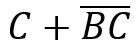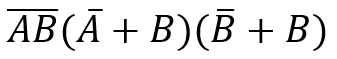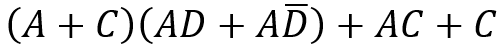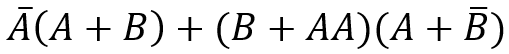Exam questions:

Категория: Boolean logic | Добавил: bzfar77 (15.09.2020)
Просмотров: 285 | | Рейтинг: 0.0/0
 Всего комментариев: 0